## Introduction

Ferroelectric materials have emerged in microelectronics as attractive candidates for data storage applications, i.e. non-volatile memory elements that retain their data when the power is turned off and that furthermore can be read, programmed and erased electrically1,2,3,4,5. In particular, interest in organic ferroelectric materials6,7,8 is growing due to their low processing temperature that allows device fabrication on foils, enabling thereby flexible electronics9,10,11,12. The most commonly used organic ferroelectric materials are poly(vinylidenefluoride) (PVDF) and its random copolymers with trifluoroethylene (P(VDF-TrFE))13,14,15. A non-volatile memory element is the ferroelectric field-effect transistor (FeFET), where the ferroelectric material is used as the gate dielectric16. Solution-processed organic FeFETs based on PVDF and P(VDF-TrFE) in combination with various semiconducting polymers and metal-oxides have been reported17,18,19,20. A reconfigurable memory array of 250 kbit has been demonstrated21.

The memory functionality is obtained by polarizing the ferroelectric gate, which modulates the charge carrier density in the semiconductor channel at the semiconductor/ferroelectric interface. Presence of compensation charges in the semiconductor is crucial for the operation of the FeFET. The two ferroelectric polarization states of the gate dielectric can only be stabilized when holes or electrons can be accumulated in the channel. Absence of these compensation charges leads to depolarization of the ferroelectric gate. Depending on the polarization state, the FeFET is programmed into a high conductive on-state or a low conductive off-state. The ratio of the on- and off-state currents, probed at zero gate bias at a low drain bias, is defined as the on/off ratio. Integration into large memory arrays requires FeFETs with well-defined on/off ratio.

In Fig. 1 a schematic of a typical transfer characteristic for a FeFET based on a p-type organic semiconductor is given in red. The threshold voltage, Vth1, is slightly positive. Hence, at zero gate bias there is current flowing between the source and drain electrodes. When the negative gate bias exceeds the coercive voltage, -Vc, the ferroelectric gate polarizes. Since the semiconductor is p-type, it can supply holes for compensation and stabilization of the negative polarization charges. As the ferroelectric polarization is much larger than the dielectric charge density, the current shows an abrupt increase at the coercive voltage. The transistor is in the on-state.

Upon scanning back the gate voltage to zero bias, the ferroelectric gate remains polarized and the current remains high. Upon further increase of the gate bias, the polarization remains stable until the positive coercive voltage, +Vc, is exceeded. The polarization of the ferroelectric gate then switches direction and positive surface charges accumulate. Since the semiconductor is p-type, it cannot provide electrons as compensating charges. Hence the ferroelectric polarization is unstable and the gate depolarizes22. As a consequence the current drops and the transistor switches to the off-state. In unipolar p-type ferroelectric transistors therefore, the ferroelectric is either negatively polarized in the on-state or depolarized in the off-state.

The current in the on-state is dominated by the ferroelectric polarization. Hence the on-state current at zero gate bias does not depend on the threshold voltage. In contrast, the off-state current strongly depends on the value of the threshold voltage. As shown in Fig. 1, the threshold voltage of the organic FeFET determines the maximum attainable on/off ratio. To achieve a high on/off ratio in a p-type FeFET, the threshold voltage should be negative. In organic field-effect transistors however, the threshold voltage is typically positive and the transistor is normally-on23,24. The value of the threshold voltage can show large device-to-device variations. In addition, the threshold voltage is influenced by the environment and by the duty cycle history25. As a result, the on/off current ratio of discrete FeFETs can show a large parameter spread.

Here we address the issue of threshold voltage spread in FeFETs. By introducing a second gate layer we gain full control over the on/off ratio. The resulting dual-gate transistor has been suggested for information storage26,27,28 Here we show that the dual-gate FeFET allows setting the threshold voltage of the transistor at the desired value by independently varying the voltages on both gates29,30. The ferroelectric gate provides the memory functionality and the second, non-ferroelectric, control gate is advantageously used to set the threshold voltage. In the resulting dual-gate FeFET the on/off ratio is optimized by the control gate. We explain the operation of a dual-gate FeFET by a quantitative analysis of the charge transport.

## Results and Discussion

### FeFET characterization

As a first step, we characterized the performance of a conventional non-ferroelectric dual-gate FET. The transfer curves were obtained by scanning the voltage on the bottom gate for various fixed top gate biases. The voltages on both gates were varied independently. The measurements are illustrated in Fig. 2a. At zero top gate bias, the device behaves as a regular field-effect transistor. The saturated source-drain current is about 1 μA and the threshold voltage is about zero volt. Figure 2a shows that the threshold voltage shifts systematically with the applied top gate bias.

The threshold voltage shift,ΔVth, can be quantified from the total charge, Qtotal, induced by the two gates: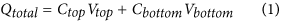where Ctop and Cbottom are the top and bottom dielectric capacitances per unit area. At the threshold voltage the total induced charge is zero. If the top gate is fixed and the bottom gate is swept, then the shift in threshold voltage is given by: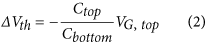where VG,top is the fixed top gate bias. Figure 2b shows the relationship between experimental shift in threshold voltage, ΔVth and VG,top. The dependence is linear and well described by Eq. (2).

Next, we characterized the performance of a dual-gate FeFET. The device layout is schematically presented in Fig. 3a. The drain bias was fixed at −20 V and the gate bias on the ferroelectric top gate was swept from −100 V to +100 V and back. The relatively high top gate bias is due to the thickness of the ferroelectric gate dielectric, which was not optimized. The high gate voltage, however, does not affect the operation mechanism. The source electrode was grounded. The transfer curves for three different control gate biases of 0 V and ±40 V are presented in Fig. 3b. First we characterize the bottom channel. We applied a zero volt top gate bias. The measured source-drain current is similar to that measured in Fig. 2a at the same bias. To characterize the top channel, we applied a zero bottom gate bias. We note that the same current is measured when using a floating bottom gate. The electrical transport can qualitatively be understood as follows. The ferroelectric switches when the coercive voltage is applied to the ferroelectric top gate, irrespective of the bias on the SiO2 control gate. The on-current at 0 V top gate bias is dominated by the ferroelectric polarization. Hence in first order approximation it is expected that the on-current does not depend on the control gate bias. In practice however, the on-current does depend on the control, bottom gate bias. The origin is partial channel depletion. The main effect of the additional control gate is a shift of the threshold voltage, as described by Eq. (2). Hence by adjusting the control gate bias, the on/off current ratio can be tuned. A phenomenological description of the transfer curves is given below. A quantitative analysis is presented in the next section.

The FeFET was characterized by applying 0 V on the SiO2 control gate. Typical transfer curves for p-type FeFETs were obtained, as shown by the black line in Fig. 3b. When the top gate bias is at −100 V, the ferroelectric is negatively polarized and the polarization is stable after removal of the gate bias due to accumulation of holes in the PTAA channel. As the gate voltage increases towards positive values, the drain current remains unchanged until about +10 V, where the polarization of the ferroelectric starts to switch direction. The channel is depleted from holes and the drain current drops. The positive polarization of the ferroelectric is not stable due to the lack of electrons in the semiconductor channel. For the back sweep, from positive to negative gate bias, the channel is depleted at the beginning; the current remains low until the threshold voltage. As the gate bias becomes lower than the threshold voltage the transistor enters the hole accumulation regime. A conducting channel is formed and current flows between the source and drain electrodes. At larger negative gate biases the ferroelectric switches and at about −70 V it is again fully polarized. We note that device optimization towards a low-voltage operation is an art in itself and beyond the scope of the present paper. Here we demonstrate the proof of concept and we discuss the device physics.

In the next step, the control gate bias is set at +40 V. The transfer curve of the FeFET is presented by the red curve in Fig. 3b. Application of a positive bias on the control SiO2 gate has two effects on the FeFET performance. Firstly, as compared to the case of zero bias on the control gate, the threshold voltage of the FeFET is shifted to the left, towards a negative value of about −20 V. Hence the off-current at 0 V gate bias decreases. Secondly, the drain current in the on-state is lower. Lowering of the on-state drain current is due to the partial depletion of the conductive channel due to the positive bias on the SiO2 control gate. Since PTAA is a p-type semiconductor, it cannot supply electrons. A positive control gate bias cannot be compensated. The control gate field is therefore unscreened and penetrates through the semiconductor layer. As a result, the top channel is electrostatically partially depleted and therefore the current is lower. Despite the lower on-state current the on/off ratio remains constant due to the lowering of the off-state current.

Next we apply −40 V on the control SiO2 gate. The transfer curve is shown as the blue line in Fig. 3b. Application of a negative bias on the control SiO2 gate has two effects on the FeFET performance. Firstly, as compared to the case of zero bias on the control gate, the threshold voltage of the FeFET is shifted to the right, towards a positive value of about +20 V. Hence the off-current at 0 V gate bias increases. Secondly, the drain current in the on-state is slightly higher due to the formation of an additional conductive channel in PTAA at the SiO2 interface. The negative bias on the control gate accumulates holes in PTAA at the SiO2 interface, which contribute to the total device current. Despite the increase in the on-state current, the on/off ratio of the FeFET is significantly decreased.

We show that the on/off current ratio is sensitive to the bias applied to the control gate. The ferroelectric gate was programmed in the on- and off-state and subsequently grounded. The corresponding state currents were recorded as a function of bias on the control gate. The on/off current ratio as a function of the bias on the control gate is presented in Fig. 4. For negative biases applied on SiO2 control gate the on/off ratio is drastically reduced. In contrast, for positive SiO2 gate biases the on/off ratio is maximized. The origin is tuning of the threshold voltage by the control gate, as described above. The large range of control gate biases at which the on/off ratio is maximum and constant makes the transistor robust to spurious external signals.

The retention of the polarization was measured to ensure that there are no adverse effects on the FeFET performance due to e.g. stress25. The ferroelectric gate was programmed either in the on- or off-state and subsequently grounded. The drain current was measured using a drain bias of −20 V and a control gate bias of +20 V. The corresponding currents as a function of time are presented in Fig. 5. The on/off ratio is constant for more than 10 hours, in good agreement with retention measurements on conventional FeFETs27,31.

We note that the channel conductance of a FeFET is controlled by switching at the source electrode32. Therefore the application of the control gate bias does not influence the switching time.

### Device physics of dual-gate FeFETs

Here we provide a quantitative analysis of the charge transport in a dual-gate FeFET. The operation is related to the capacitances of the gate dielectrics and of the semiconductor layer. Assuming a parallel plate capacitor, we calculated Cferro = 8.85 nF/cm2 for the P(VDF-TrFE) layer (ε = 10), Csemi = 53 nF/cm2 for the PTAA semiconductor layer (ε = 3) and Ccontrol = 3.5 nF/cm2 for the SiO2 layer (ε = 3.9). We first consider the case of a fixed negative bias on the bottom, control gate. An accumulation layer is formed at the control gate dielectric/semiconductor interface. Starting with a depolarized ferroelectric gate, the device is simply a conventional dual-gate transistor. The threshold voltage shift is given by: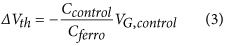comparable to Eq. (2). At a VG, control of −40 V, the threshold voltage shift of the dual gate FeFET transistor is calculated as about 16 V, in good agreement with the experimentally observed value of about 15 V, as seen in Fig. 3b. Therefore, at a VG, ferro of 0 V the off-state current of the dual-gate FeFET is increased.

On the other hand, when the ferroelectric gate is polarized in the on-state, a second accumulation layer is formed at the ferroelectric/semiconductor interface. The ferroelectric gate remains fully polarized at a VG, ferro of 0 V. The control gate field is fully screened by the bottom accumulation channel. Both channels are independent. The total on-state current of the dual-gate FeFET is the sum of the currents flowing in each channel. Despite this slightly higher current in the on-state, the on/off ratio of the dual-gate FeFET is lowered due to the increased off-state current.

We now consider the case when the control gate is kept at fixed positive bias. Since PTAA is a p-type semiconductor, it cannot supply electrons. Thus when a positive gate bias is applied on the control gate, the bottom-channel is depleted. Starting with the depolarized off-state of the ferroelectric top-gate, the device is again a dual gate transistor. The depleted part of the semiconductor forms a capacitor in series with the control gate dielectric. Then, in Eq. (3) Ccontrol has to be replaced by: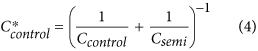The threshold voltage shift at a VG, control of +40 V, is then calculated to be about −15 V, in good agreement with the experimentally observed value of about −20 V, as shown in Fig. 3. The threshold voltage of the dual-gate FeFET is now negative, which implies that at a VG, ferro of 0 V, the off-state current that is running between the electrodes is only the leakage current, here 1–2 nA.

We now calculate the current in the on-state of the dual-gate FeFET. Due to the positive gate bias on the control gate the bottom-channel is depleted. Therefore the bottom-gate field is unscreened and penetrates through the semiconductor layer, electrostatically affecting the top channel. The ferroelectric is fully polarized. The polarization charge is compensated for by charge carriers in the channel and by the unscreened control gate field. The amount of charges in the semiconductor channel can be estimated as follows.

In a circuit consisting of a ferroelectric capacitor, Cferro, in series with a linear capacitor, Cs, Black et al.33 have shown that the charge over the ferroelectric capacitor, Qferro, is equal to: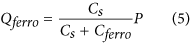where P is the polarization. To describe a dual-gate FeFET, we extend the analysis of Black et al. by including the additional control gate. The equivalent circuit is shown in Fig. 6. We assume that the ferroelectric remains fully polarized. The ferroelectric gate, VG, ferro, is grounded. The voltage over the ferroelectric, Vf, plus the voltage drop over the depleted semiconductor/control gate dielectric stack, Vo, equals the applied control gate bias, VG, control: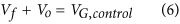The central node of the circuit is electrically neutral, meaning that the charge at the ferroelectric, Qferro, equals the charge over the semiconductor/control gate dielectric stack, Qo. Eq. (6) becomes: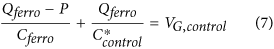where Qferro-P / Cferro is the voltage over the ferroelectric and P is the remanent polarization. For Qferro we then obtain: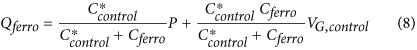When the control gate is grounded, Eq. (8) reduces to Eq. (5), as derived in reference 33. For a control gate bias of +40 V and a remanent polarization of 7 μC/cm2 we obtain a value of 2 μC/cm2 for Qferro.

In the derivation we have treated the semiconductor as a pure insulator. In reality the polarized ferroelectric gate induces a charge density of 7 μC/cm2 in the semiconductor channel. The calculated Qferro corresponds to the depleted charge density due to the presence of the unscreened control gate. The charge carrier density in the channel amounts to the polarization-induced charge minus Qferro. For the present case of a control gate bias of +40 V, we calculate a reduction of 30% in the charge carrier density.

The charge carrier density reduction can also be directly calculated from the on-state current. Since the current between source and drain has a linear relationship with charge density, the on-state current therefore should reach 70% of its original value when the control gate is grounded. Fig. 3b shows that the on-state currents are 1.1 × 10−6 A and 7.5 × 10−7 A for VG, control of 0 V and +40 V, respectively. The ratio between these currents is 69%, in good agreement with the estimated 70%, as calculated above.

### Summary and Conclusion

In summary, we have investigated the device physics of a dual-gate FeFET. The transistors comprised a ferroelectric gate of P(VDF-TrFE) and an SiO2 control gate. The ferroelectric gate provides the memory functionality and the non-ferroelectric, control gate, is used to fine tune the position of the threshold voltage. The on/off ratio could thus be maximized at the readout bias. We related the operation to the capacitances of the gate dielectrics and of the semiconductor layer. The charge transport in a dual-gate FeFET could be quantitatively interpreted.

## Methods

Dual-gate FeFETs were fabricated on heavily doped n-type Si monitor wafers that act as a common control gate. The gate dielectric is a 1 μm thermally grown SiO2 oxide layer. We note that SiO2 can be replaced by organic gate dielectrics, which are a prerequisite for practical applications. However, here we are only interested in the operation mechanism of discrete dual-gate FeFETs. Gold source and drain electrodes, 50 nm thick, were defined by photolithography using a 2 nm Ti adhesion layer. The SiO2 layer was passivated with hexamethyldisilazane prior to semiconductor deposition. Polytriarylamine (PTAA) films with a layer thickness of approximately 80 nm were spin-coated from toluene. On the PTAA, the ferroelectric gate dielectric P(VDF-TrFE) (80/20 mol %) was spin-coated from butanone. The P(VDF-TrFE) layer thickness was measured with a Dektak profilometer and amounted to 1 μm. Subsequently the transistor was annealed at 140 °C in vacuum to enhance the crystallinity of the P(VDF-TrFE) layer. The top gate Au electrode was evaporated through a shadow mask. Using the same device fabrication recipe, dual-gate field-effect transistors with CYTOPTM as a non-ferroelectric top gate, were also fabricated. Electrical measurements were performed in vacuum and in ambient conditions using an Agilent HP4155C semiconductor parameter analyser.